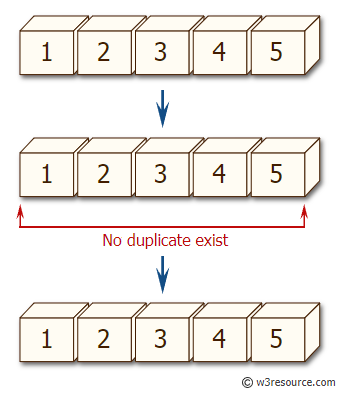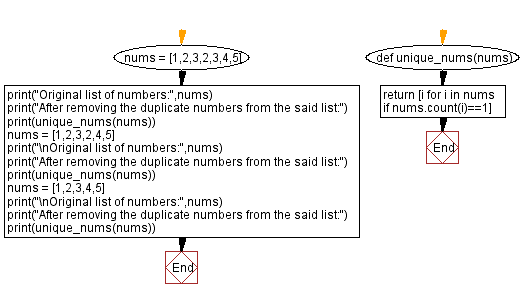﻿ Python: Remove the duplicate numbers from a given list of numbers - w3resource# Python: Remove the duplicate numbers from a given list of numbers

## Python Basic - 1: Exercise-110 with Solution

Write a Python program to remove the duplicate numbers from a given list of numbers.

Sample Solution:

Python Code:

``````def unique_nums(nums):
return [i for i in nums if nums.count(i)==1]
nums = [1,2,3,2,3,4,5]
print("Original list of numbers:",nums)
print("After removing the duplicate numbers from the said list:")
print(unique_nums(nums))
nums = [1,2,3,2,4,5]
print("\nOriginal list of numbers:",nums)
print("After removing the duplicate numbers from the said list:")
print(unique_nums(nums))
nums = [1,2,3,4,5]
print("\nOriginal list of numbers:",nums)
print("After removing the duplicate numbers from the said list:")
print(unique_nums(nums))
``````

Sample Output:

```Original list of numbers: [1, 2, 3, 2, 3, 4, 5]
After removing the duplicate numbers from the said list:
[1, 4, 5]

Original list of numbers: [1, 2, 3, 2, 4, 5]
After removing the duplicate numbers from the said list:
[1, 3, 4, 5]

Original list of numbers: [1, 2, 3, 4, 5]
After removing the duplicate numbers from the said list:
[1, 2, 3, 4, 5]
```

Pictorial Presentation:Flowchart:Python Code Editor:

Have another way to solve this solution? Contribute your code (and comments) through Disqus.

What is the difficulty level of this exercise?

Test your Programming skills with w3resource's quiz.

﻿

## Python: Tips of the Day

What is the difference between Python's list methods append and extend?

append: Appends object at the end.

```x = [1, 2, 3]
x.append([4, 5])
print (x)
```

Output:

```[1, 2, 3, [4, 5]]
```

extend: Extends list by appending elements from the iterable.

```x = [1, 2, 3]
x.extend([4, 5])
print (x)
```

Output:

```[1, 2, 3, 4, 5]
```

Ref: https://bit.ly/2AZ6ZFq